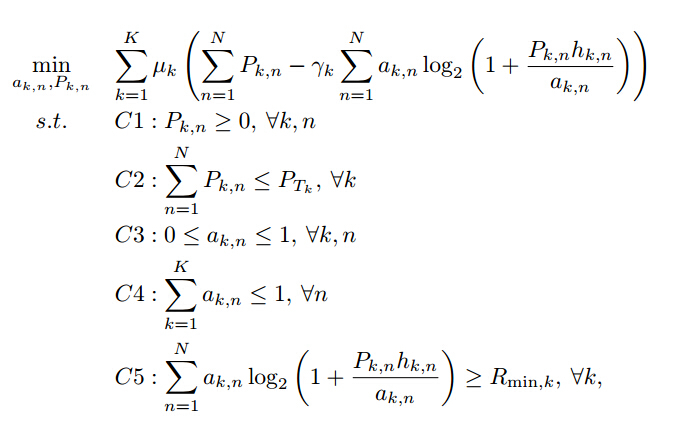# How to make this convex optimization be accepted by CVX?

Recently, I have a problem about using CVX to solve the convex problem as follows,. In this problem, the denotations except for a_{k,n} and P_{k,n} are all constants. I have proved this program is a convex one. But I have no idea about its realization on CVX. So, I look for help here.

I have known that -rel_entr(a_{k,n},a_{k,n}+h_{k,n}P_{k,n}) is helpful to let this program be accepted by CVX. However, I still have no idea of the deal on double summation.

Have you tried just entering a double, i.e., nested, sum just as you would in standard MATLAB?